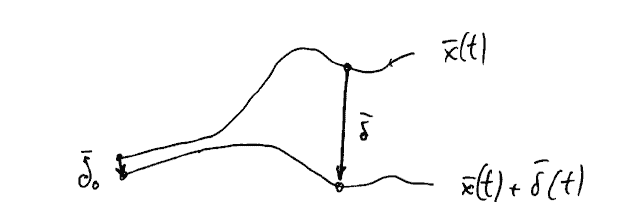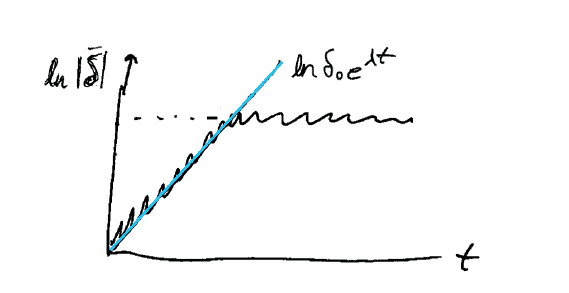The Lorenz system was presented in the previous lecture. The following slides present an overview of selected properties of the system. The bifurcation analysis is shown for varied $r$ while keeping the other parameters constant.

## Properties of Lorenz attractor

Lorenz attractor has the form
$$\left{\begin{array}{l} \dot{x}=d(y-x) \ \dot{y}=r x-y-x z \ \dot{z}=x y-b z \end{array}\right.$$
where $r, d$, and $b$ are the parameters. For $r=28, d=10$, and $b=8 / 3$, the Lorenz system has the chaotic solution. Other parameter values may generate other types of solutions.
Lorenz equations also arise in simplified models for lasers, dynamos, thermosyphons, brushless DC motors, electric circuits, chemical reactions and forward osmosis.

• There exists a symmetric pair of solutions. If $(x, y) \rightarrow(-x,-y)$ the system stays the same. If solution $(x(t), y(t), z(t))$ exists then solution $(-x(t),-y(t), z(t))$ is also a solution.
• Lorenz system is dissipative $^{1}$ : volumes in phase space contract under the flow. As $t \rightarrow \infty, V \rightarrow 0$
$$\begin{array}{c} \nabla \cdot \dot{\vec{x}}=-(d+1+b)<0=\text { const. } \ \dot{V}=-(d+1+b) V \end{array}$$

## Bifurcation analysis of Lorenz attractor

For $r<1(d=10, b=8 / 3)$ there is only one stable fixed point located at the origin. This point corresponds to no convection. All orbits converge to the origin – a global attractor. A supercritical pitchfork bifurcation occurs at $r=1$, and for $r>1$ two additional fixed points appear at
$$\left(x^{}, y^{}, z^{*}\right)=C^{\pm}=(\pm \sqrt{\beta(r-1)}, \pm \sqrt{\beta(r-1)}, r-1)$$
These correspond to steady convection. Fixed points are stable only if
$$rb+1. At a critical value r=r_{\text {Hopf }}, both stable fixed points lose stability through a subcritical Hopf bifurcation. As we decrease r from r_{\text {Hopf }}, the unstable limit cycles expand and pass precariously close to the saddle point at the origin. At r=13.926 the cycles touch the saddle point and become homoclinic orbits; hence we have a homoclinic bifurcation which is referred to as the first homoclinic explosion. Below r=13.926 there are no limit cycles. The region 13.926<r<24.06 is referred to as transient chaos ^{2} region. Here, the chaotic trajectories eventually settle at C^{+} or C^{-}. For r>24.06 and r>r_{\text {Hopf }}=28 (immediate vicinity): no stable limit-cycles exist; trajectories do not escape to infinity (dissipation); do not approach an invariant torus (quasi-periodicity). Almost all initial conditions (I.C.s) will tend to an invariant set - the Lorenz attractor – a strange attractor and a fractal. Note: No quasi-periodic solutions for r>r_{\text {Hopf }} are possible because of the dissipative property of the flow. For r \gg r_{\text {Hopf }} different types of chaotic dynamics exist e.g. noisy periodicity, transient and intermittent chaos. One can even find transient chaos settling to periodic orbits ^{3}.Rapidly diverging trajectories where the initial separation \left|\vec{\delta}{0}\right|=\delta{0} \approx 0. ## Lyapunov exponents From numerical integration of the Lorenz system we know that the motion on the attractor exhibits sensitive dependence on initial conditions. This means that two trajectories starting very close to each other will rapidly diverge, and thereafter have totally different futures It can be demonstrated that after the transient behaviour has elapsed and a trajectory has settled on the attracting set (Lorenz attractor) the norm of the separation vector where in the case of Lorenz system λ ≈ 0.9. Hence, neighbouring trajectories diverge exponentially fast. Equivalently, if we plot ln |󰂓 δ | vs. t, a logarithmic plot, we find a curve that is close to a straight line with a positive slope of λ,Norm of separation vector |\vec{\delta}| as a function of t. Slope \lambda is shown with the straight blue line. The exponential divergence must stop when the separation is comparable to the diameter of the attractor (attracting set, think Lorenz butterfly) - the trajectories obviously can’t get any farther apart than that. This explains the levelling off or saturation of the curve \ln |\vec{\delta}(t)| The exponent \lambda is often called the Lyapunov exponent, although this is a sloppy use of the term, for two reasons: First, there are actually n different Lyapunov exponents for each degree of freedom of an n-dimensional system (λx, λy, λz, . . .). Second, λ depends slightly on which trajectory we study. We should average over many trajectories (initial conditions) and over many different points on the same trajectory to get the true value of λ. Lyapunov exponent λ of a system is usually close to the biggest of the exponents related to the degrees of freedom (λx, λy, λz, . . .). The biggest exponent governs the resulting slope shown in Figs. 2 and 3. Note: λ > 0 is a signature of chaos ## Kolmogorov entropy Also known as the metric entropy, Kolmogorov-Sinai entropy, or KS entropy. The Kolmogorov entropy is zero for non-chaotic motion and positive for chaotic motion. For \lambda_{i}>0 one can find the Kolmogorov entropy using the following formula:$$
K=\sum_{i} \lambda_{i}

where $i$ spans the number of degrees of freedom of a given chaotic system. Kolmogorov entropy is a measure of the growth of uncertainty due to the expansion rate given by the positive Lyapunov exponents $\lambda_{i}$.

Categories: 数学代写